This vignette details the use of energy-based detection in ohun. The energy detector approach uses amplitude envelopes to infer the position of sound events. Amplitude envelopes are representations of the variation in energy through time. This type of detector doesn’t require highly stereotyped sound events, although they work better on high quality recordings in which the amplitude of target sound events is higher than the background noise (i.e. high signal-to-noise ratio):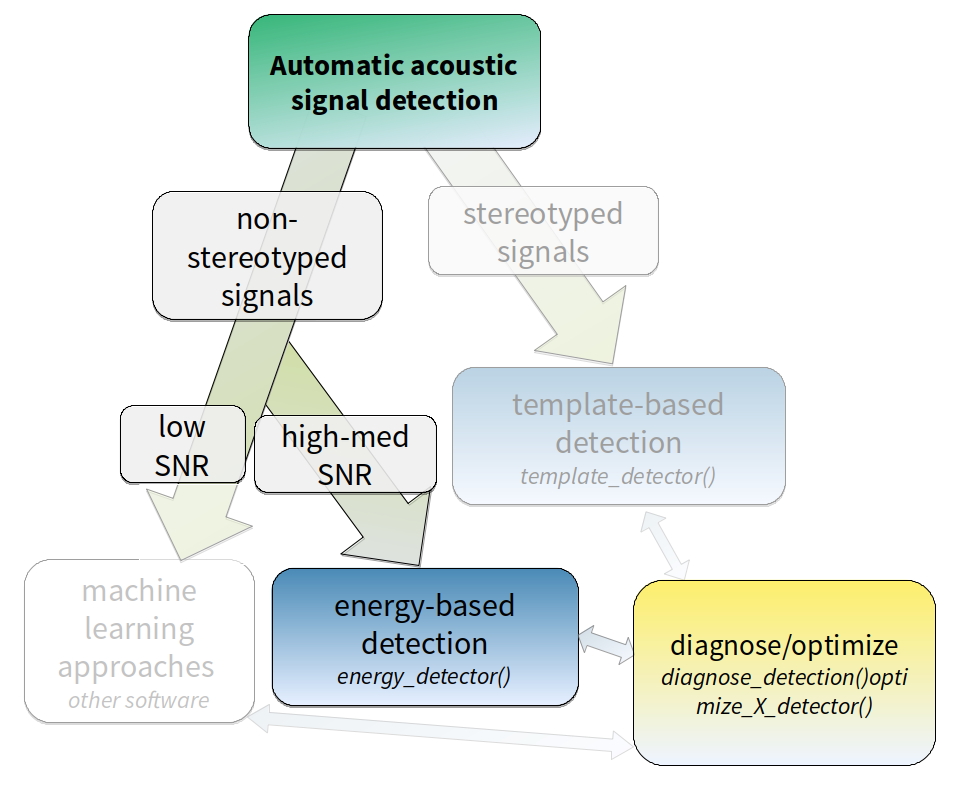Diagram depicting how target sound event features can be used to tell the most adequate sound event detection approach. Steps in which ‘ohun’ can be helpful are shown in color. (SNR = signal-to-noise ratio)

First, we need to install the package. It can be installed from CRAN as follows:

``````# From CRAN would be
install.packages("ohun")

library(ohun)``````

To install the latest developmental version from github you will need the R package remotes:

``````# install package
remotes::install_github("maRce10/ohun")

library(ohun)
library(tuneR)
library(warbleR)``````

The package comes with an example reference table containing annotations of long-billed hermit hummingbird songs from two sound files (also supplied as example data: ‘lbh1’ and ‘lbh2’), which will be used in this vignette. The example data can be load and explored as follows:

``````# load example data
data("lbh1", "lbh2", "lbh_reference")

# save sound files
tuneR::writeWave(lbh1, file.path(tempdir(), "lbh1.wav"))
tuneR::writeWave(lbh2, file.path(tempdir(), "lbh2.wav"))

# select a subset of the data
lbh1_reference <-
lbh_reference[lbh_reference\$sound.files == "lbh1.wav",]

# print data
lbh1_reference``````
`` [30mObject of class  [1m'selection_table' [22m [39m``
`` [90m* The output of the following call: [39m``
`` [90m [3m`[.selection_table`(X = lbh_reference, i = lbh_reference\$sound.files ==  [23m [39m [90m [3m"lbh1.wav") [23m [39m``
`````` [90m [1m
Contains: [22m *  A selection table data frame with 10 rows and 6 columns: [39m``````
`` [90m|   |sound.files | selec|  start|    end| bottom.freq| top.freq| [39m``
`` [90m|:--|:-----------|-----:|------:|------:|-----------:|--------:| [39m``
`` [90m|10 |lbh1.wav    |    10| 0.0881| 0.2360|      1.9824|   8.4861| [39m``
`` [90m|11 |lbh1.wav    |    11| 0.5723| 0.7202|      2.0520|   9.5295| [39m``
`` [90m|12 |lbh1.wav    |    12| 1.0564| 1.1973|      2.0868|   8.4861| [39m``
`` [90m|13 |lbh1.wav    |    13| 1.7113| 1.8680|      1.9824|   8.5905| [39m``
`` [90m|14 |lbh1.wav    |    14| 2.1902| 2.3417|      2.0520|   8.5209| [39m``
`` [90m|15 |lbh1.wav    |    15| 2.6971| 2.8538|      1.9824|   9.2513| [39m``
`` [90m... and 4 more row(s) [39m``
`````` [90m
* A data frame (check.results) generated by check_sels() (as attribute)  [39m``````
`` [90mcreated by warbleR < 1.1.21 [39m``

We can plot the annotations on top of the spectrogram and amplitude envelope to further explore the data (this function only plots one wave object at the time, not really useful for long files):

``````# print spectrogram
label_spectro(wave = lbh1, reference = lbh1_reference, hop.size = 10, ovlp = 50, flim = c(1, 10), envelope = TRUE)``````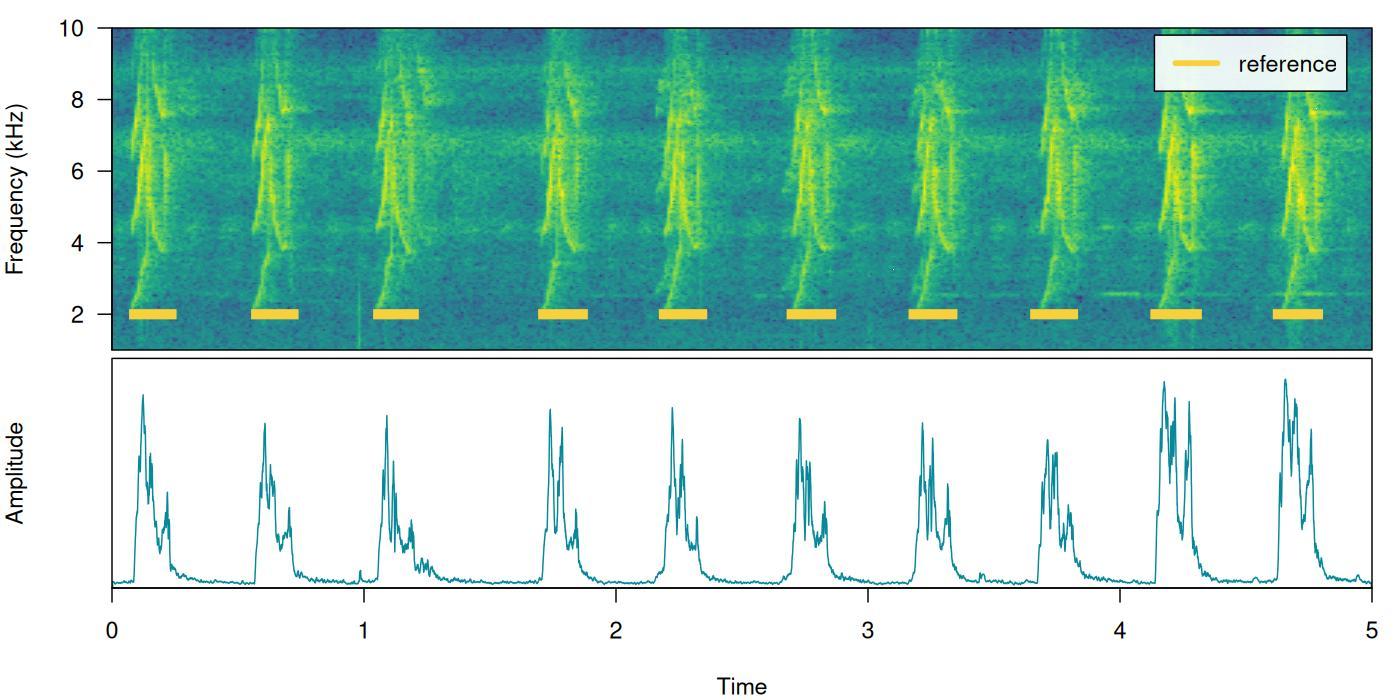## How it works

The function `ernergy_detector()` performs this type of detection. We can understand how to use `ernergy_detector()` using simulated sound events. We will do that using the function `simulate_songs()` from warbleR. In this example we simulate a recording with 10 sounds with two different frequency ranges and durations:

``````# install this package first if not installed
# install.packages("Sim.DiffProc")

#Creating vector for duration
durs <- rep(c(0.3, 1), 5)

#Creating simulated song
set.seed(12)
simulated_1 <-
warbleR::simulate_songs(
n = 10,
durs = durs,
freqs = 5,
sig2 = 0.01,
gaps = 0.5,
harms = 1,
bgn = 0.1,
path = tempdir(),
file.name = "simulated_1",
selec.table = TRUE,
shape = "cos",
fin = 0.3,
fout = 0.35,
samp.rate = 18
)\$wave``````

The function call saves a ‘.wav’ sound file in a temporary directory (`tempdir()`) and also returns a `wave` object in the R environment. This outputs will be used to run energy-based detection and creating plots, respectively. This is how the spectrogram and amplitude envelope of the simulated recording look like:

``````# plot spectrogram and envelope
label_spectro(wave = simulated_1,
env = TRUE,
fastdisp = TRUE)``````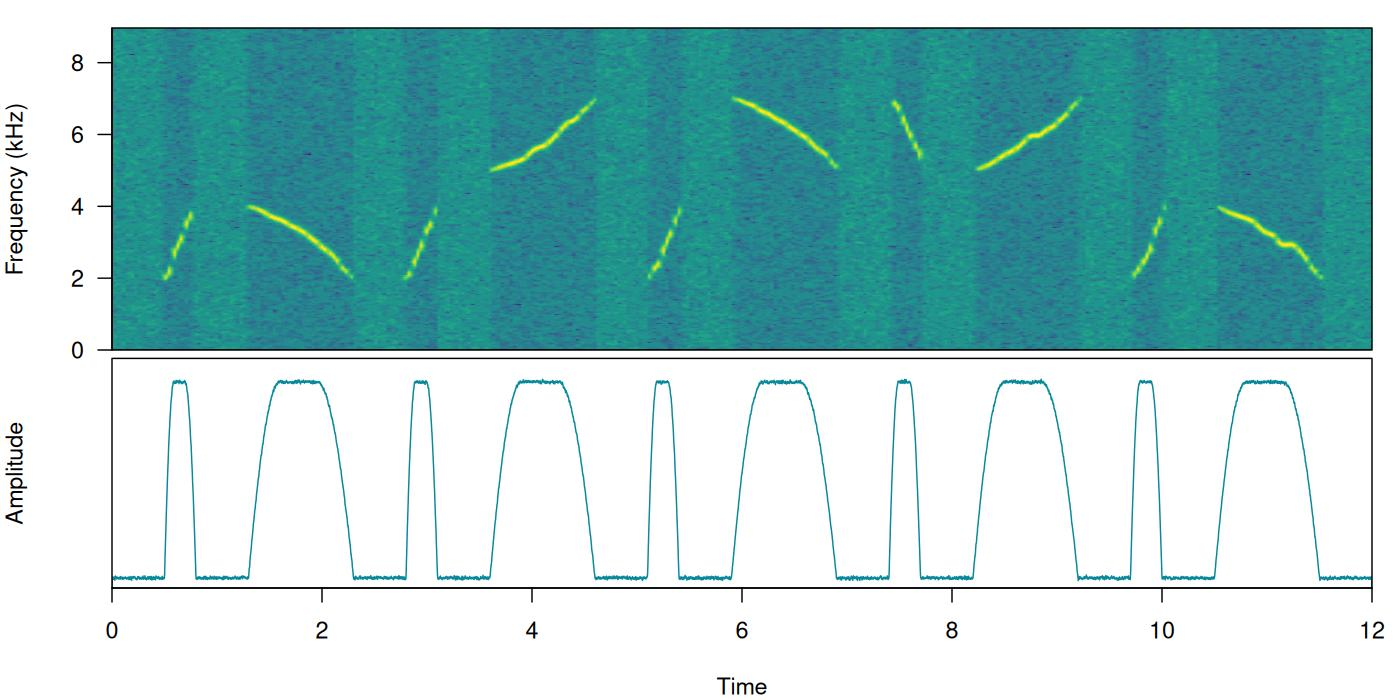Note that the amplitude envelope shows a high signal-to-noise ratio of the sound events, which is ideal for energy-based detection. This can be conducted using `ernergy_detector()` as follows:

``````# run detection
detection <-
energy_detector(
files = "simulated_1.wav",
bp = c(2, 8),
threshold = 50,
smooth = 150,
path = tempdir()
)

# plot spectrogram and envelope
label_spectro(
wave = simulated_1,
envelope = TRUE,
detection = detection,
threshold = 50
)``````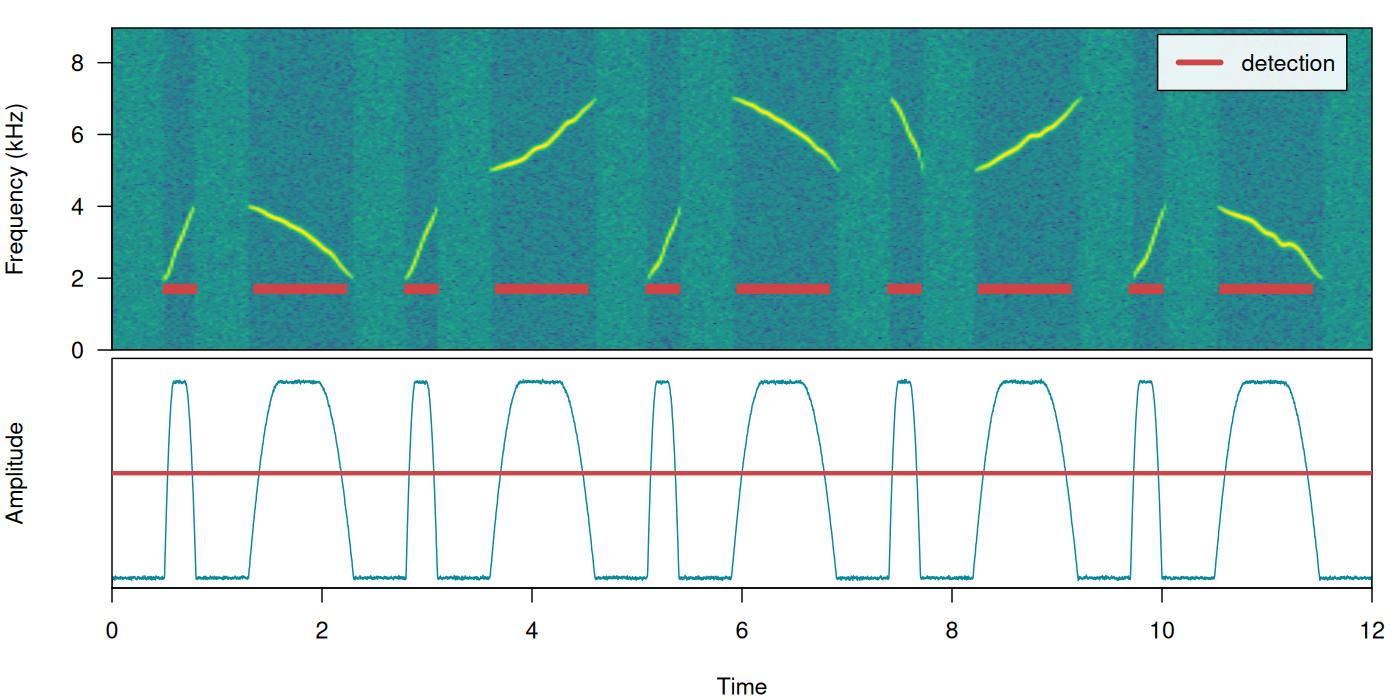The output is a selection table:

``detection``
`` [30mObject of class  [1m'selection_table' [22m [39m``
`` [90m* The output of the following call: [39m``
`` [90m [3menergy_detector(files = "simulated_1.wav", path = tempdir(),  [23m [39m [90m [3mbp = c(2, 8), smooth = 150, threshold = 50) [23m [39m``
`````` [90m [1m
Contains: [22m *  A selection table data frame with 10 rows and 5 columns: [39m``````
`` [90m|sound.files     | duration| selec|  start|    end| [39m``
`` [90m|:---------------|--------:|-----:|------:|------:| [39m``
`` [90m|simulated_1.wav |   0.2328|     1| 0.5309| 0.7638| [39m``
`` [90m|simulated_1.wav |   0.7947|     2| 1.3955| 2.1901| [39m``
`` [90m|simulated_1.wav |   0.2334|     3| 2.8308| 3.0642| [39m``
`` [90m|simulated_1.wav |   0.7944|     4| 3.6955| 4.4899| [39m``
`` [90m|simulated_1.wav |   0.2333|     5| 5.1307| 5.3641| [39m``
`` [90m|simulated_1.wav |   0.7945|     6| 5.9956| 6.7901| [39m``
`` [90m... and 4 more row(s) [39m``
`````` [90m
* A data frame (check.results) generated by check_sels() (as attribute)  [39m``````
`` [90mcreated by warbleR 1.1.29 [39m``

Now we will make use of some additional arguments to filter out specific sound events based on their structural features. For instance we can use the argument `minimum.duration` to provide a time treshold (in ms) to exclude short sound events and keep only the longest sound events:

``````# run detection
detection <-
energy_detector(
files = "simulated_1.wav",
bp = c(1, 8),
threshold = 50,
min.duration = 500,
smooth = 150,
path = tempdir()
)

# plot spectrogram
label_spectro(wave = simulated_1, detection = detection)``````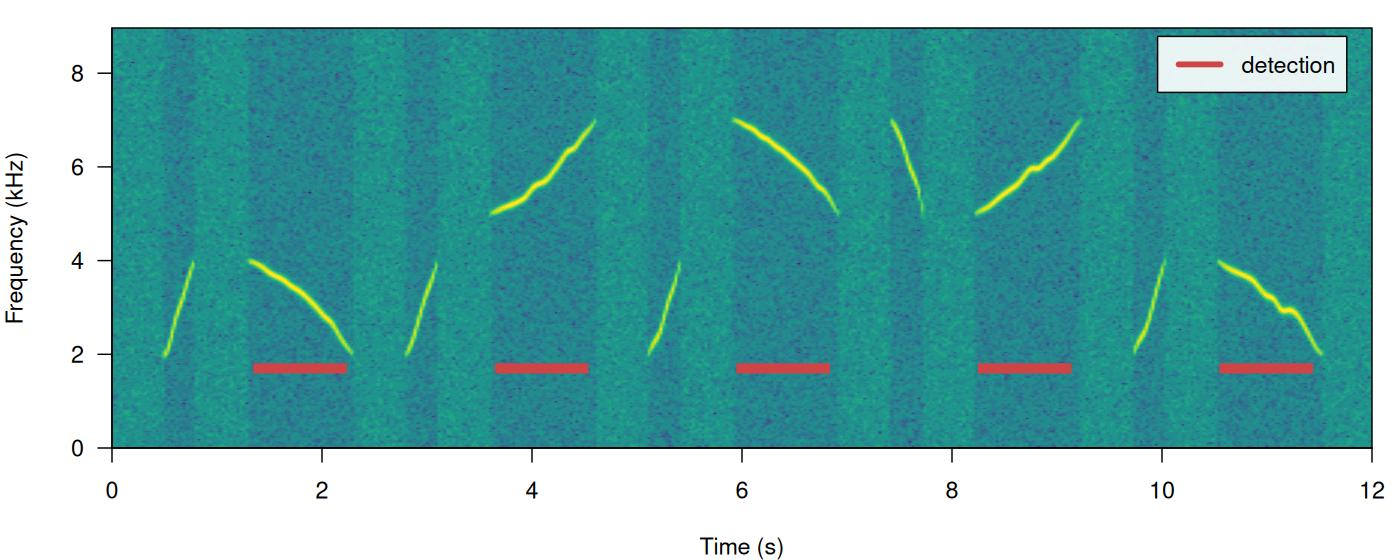We can use the argument `max.duration` (also in ms) to exclude long sound events and keep the short ones:

``````# run detection
detection <- energy_detector(files = "simulated_1.wav", bp = c(1, 8),  threshold = 50, smooth = 150, max.duration = 500, path = tempdir())

# plot spectrogram
label_spectro(wave = simulated_1,  detection = detection)``````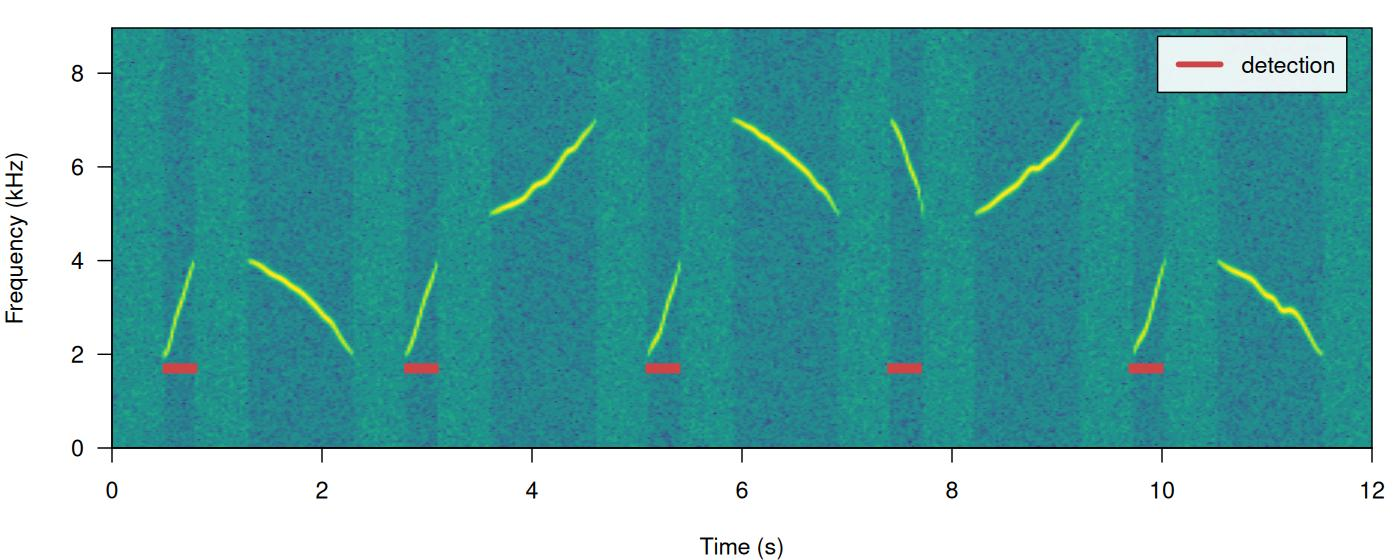We can also focus the detection on specific frequency ranges using the argument `bp` (bandpass). By setting `bp = c(5, 8)` only those sound events found within that frequency range (5-8 kHz) will be detected, which excludes sound events below 5 kHz:

``````# Detecting
detection <- energy_detector(files = "simulated_1.wav", bp = c(5, 8), threshold = 50, smooth = 150, path = tempdir())

# plot spectrogram
label_spectro(wave = simulated_1,  detection = detection)``````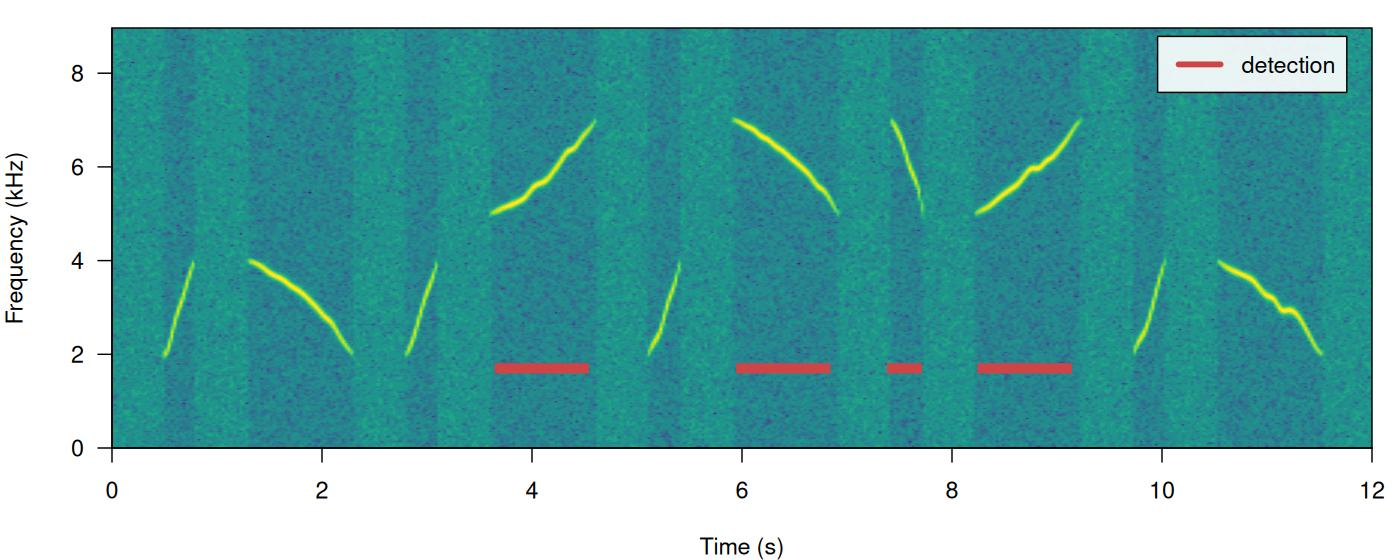The same logic can be applied to detect those sound events found below 5 kHz. We just need to set the upper bound of the band pass filter below the range of the higher frequency sound events (for instance `bp = (0, 6)`):

``````# Detect
detection <-
energy_detector(
files = "simulated_1.wav",
bp = c(0, 6),
threshold = 50,
min.duration = 1,
smooth = 150,
path = tempdir()
)

# plot spectrogram
label_spectro(wave = simulated_1,  detection = detection)``````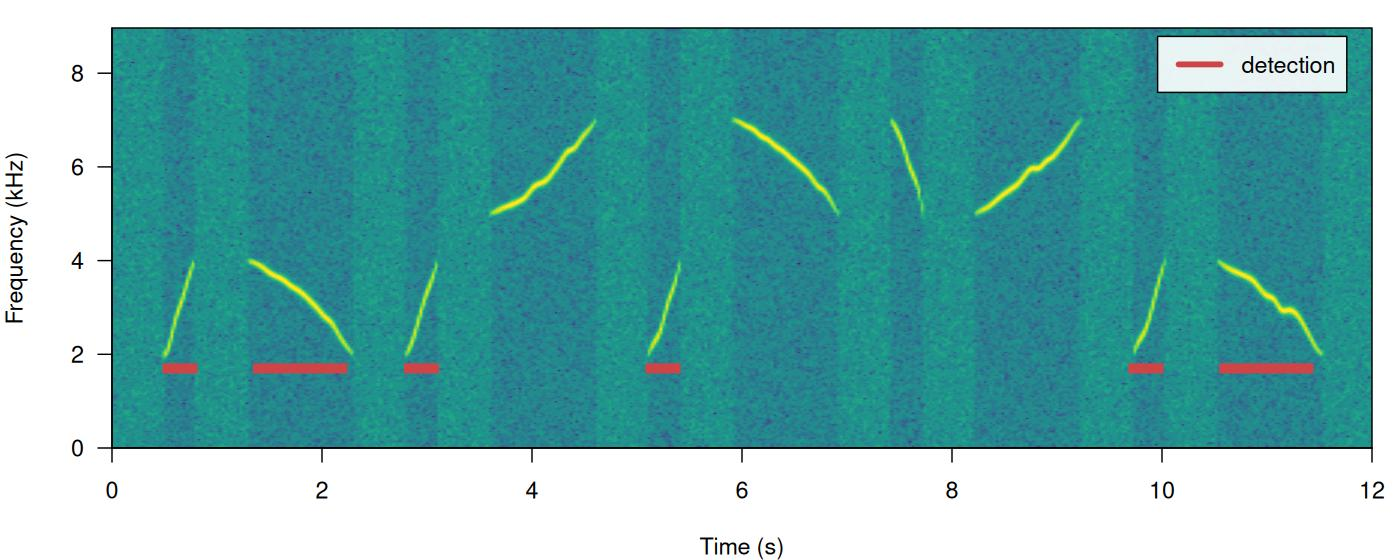Amplitude modulation (variation in amplitude across a sound event) can be problematic for detection based on amplitude envelopes. We can also simulate some amplitude modulation using `warbleR::simulate_songs()`:

``````#Creating simulated song
set.seed(12)

#Creating vector for duration
durs <- rep(c(0.3, 1), 5)

sim_2 <-
sim_songs(
n = 10,
durs = durs,
freqs = 5,
sig2 = 0.01,
gaps = 0.5,
harms = 1,
bgn = 0.1,
path = tempdir(),
file.name = "simulated_2",
selec.table = TRUE,
shape = "cos",
fin = 0.3,
fout = 0.35,
samp.rate = 18,
am.amps = c(1, 2, 3, 2, 0.1, 2, 3, 3, 2, 1)
)

# extract wave object and selection table
simulated_2 <- sim_2\$wave
sim2_sel_table <- sim_2\$selec.table

# plot spectrogram
label_spectro(wave = simulated_2, envelope = TRUE)``````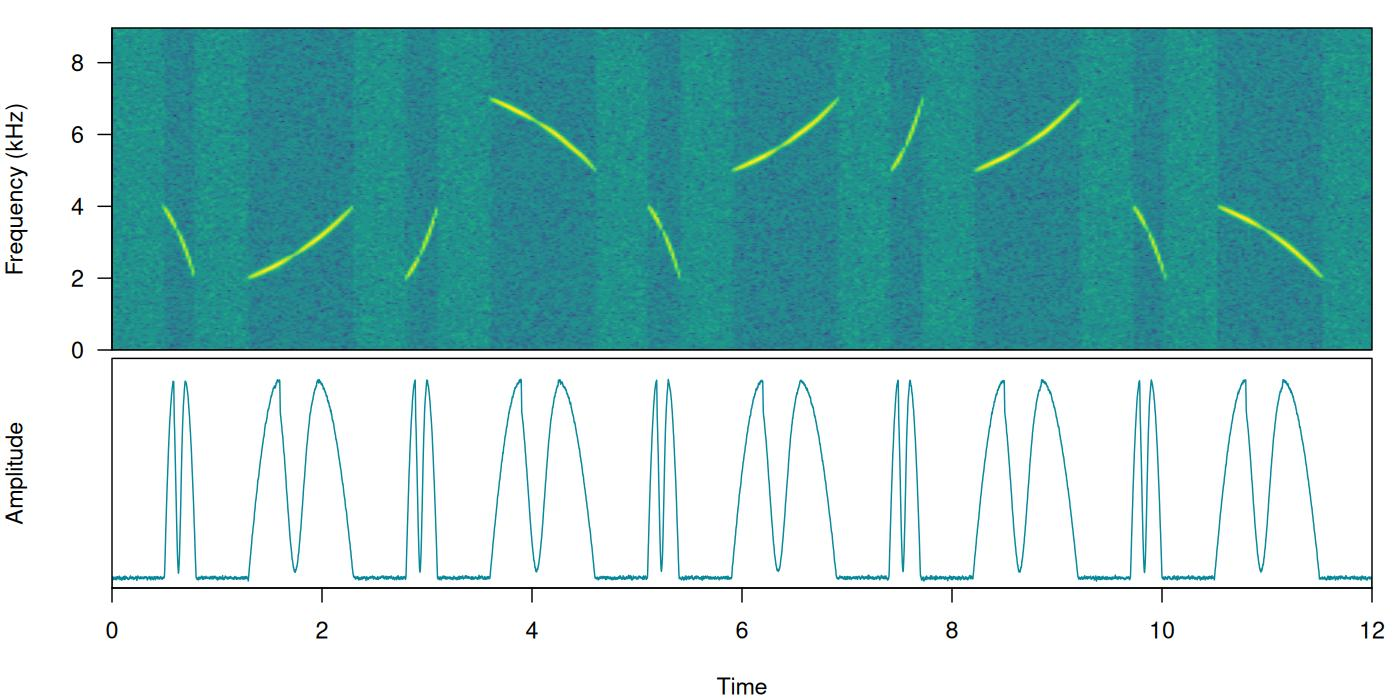When sound events have strong amplitude modulation they can be split during detection:

``````# detect sounds
detection <- energy_detector(files = "simulated_2.wav", threshold = 50, path = tempdir())

# plot spectrogram
label_spectro(wave = simulated_2, envelope = TRUE, threshold = 50, detection = detection)``````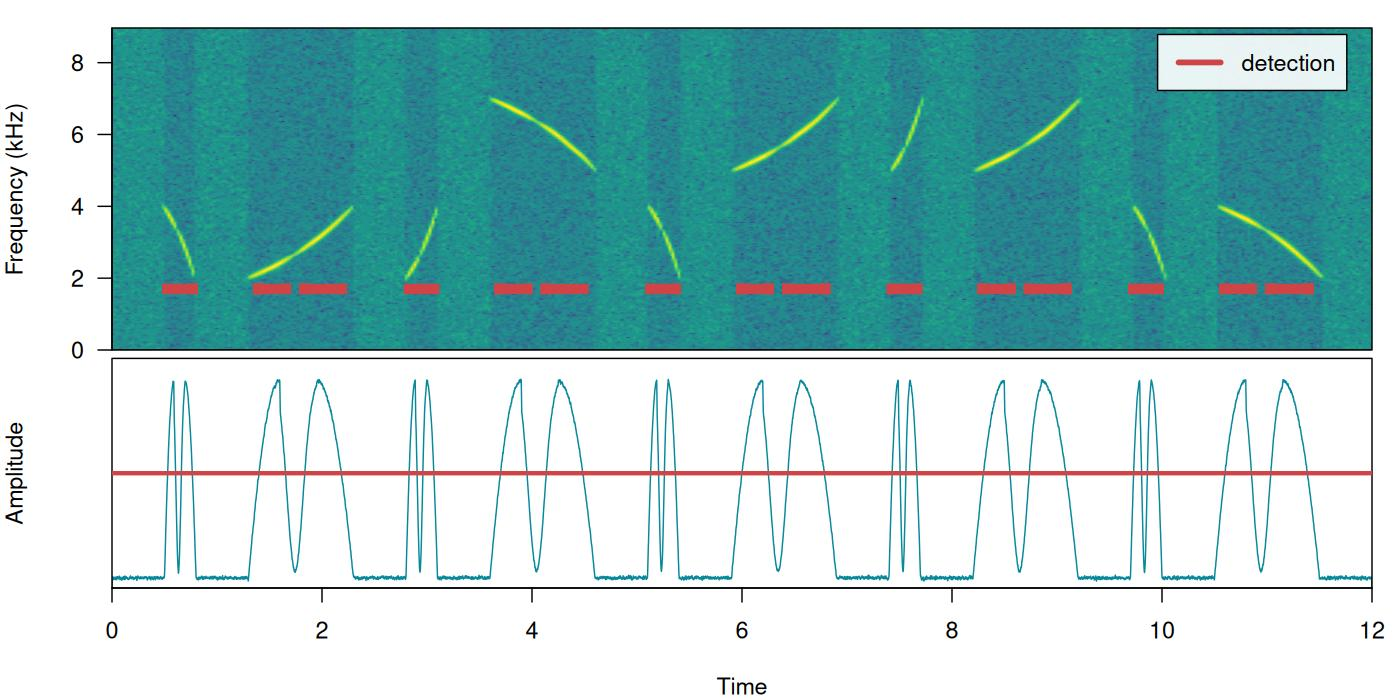There are two arguments that can deal with this: `holdtime` and `smooth`. `hold.time` allows to merge split sound events that are found within a given time range (in ms). This time range should be high enough to merge things belonging to the same sound event but not too high so it merges different sound events. For this example a `hold.time` of 200 ms can do the trick (we know gaps between sound events are ~0.5 s long):

``````# detect sounds
detection <-
energy_detector(
files = "simulated_2.wav",
threshold = 50,
min.duration = 1,
path = tempdir(),
hold.time = 200
)

# plot spectrogram
label_spectro(
wave = simulated_2,
envelope = TRUE,
threshold = 50,
detection = detection
)``````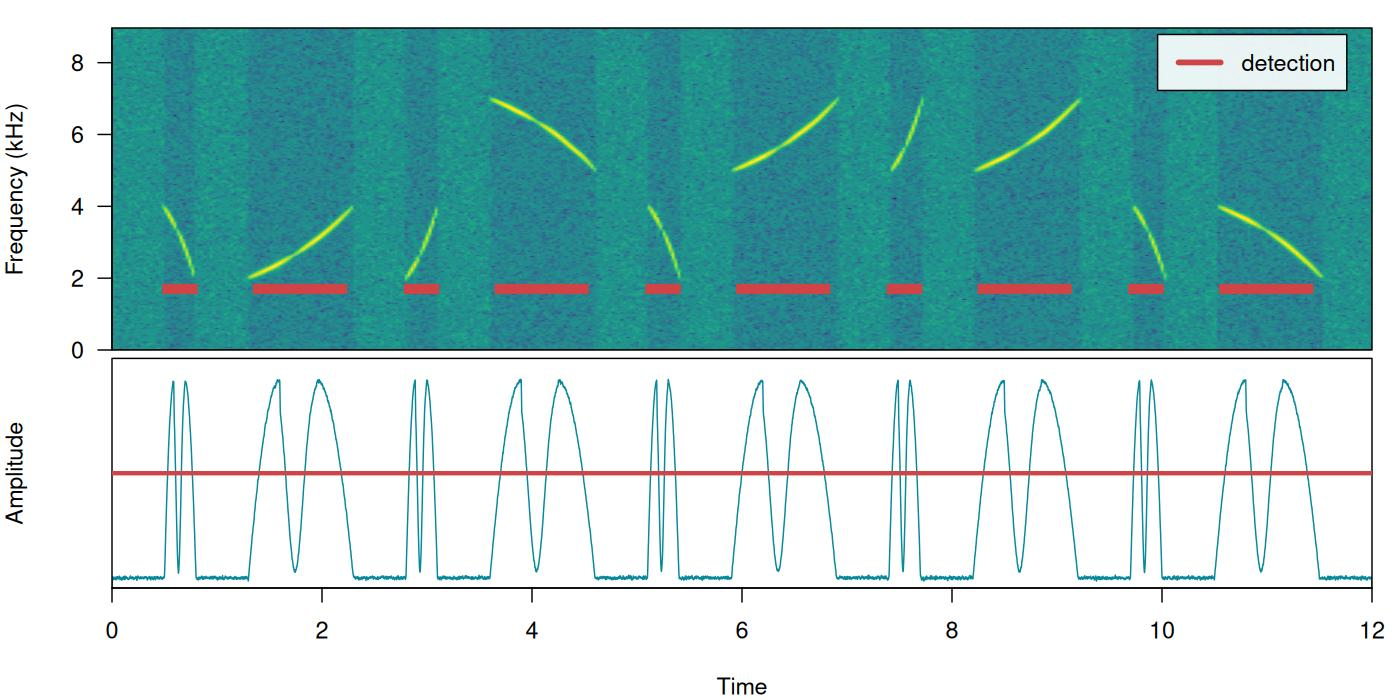`smooth` works by merging the amplitude envelope ‘hills’ of the split sound events themselves. It smooths envelopes by applying a sliding window averaging of amplitude values. It’s given in ms of the window size. A `smooth` of 350 ms can merged back split sound events from our example:

``````# detect sounds
detection <-
energy_detector(
files = "simulated_2.wav",
threshold = 50,
min.duration = 1,
path = tempdir(),
smooth = 350
)

# plot spectrogram
label_spectro(
wave = simulated_2,
envelope = TRUE,
threshold = 50,
detection = detection,
smooth = 350
)``````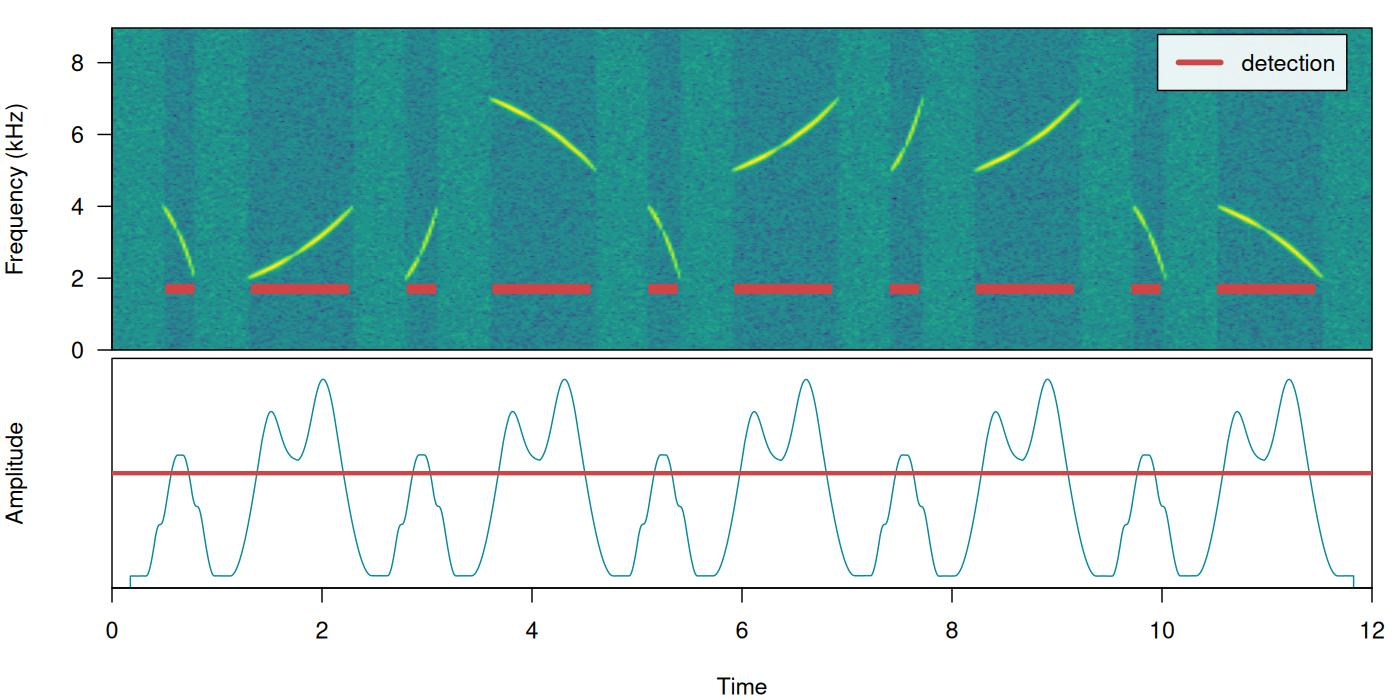The function has some additional arguments for further filtering detections (`peak.amplitude`) and speeding up analysis (`thinning` and `parallel`).

## Optimizing energy-based detection

This last example using `smooth` can be used to showcase how the tunning parameters can be optimized. As explained above, to do this we need a reference table that contains the time position of the target sound events. The function `optimize_energy_detector()` can be used finding the optimal parameter values. We must provide the range of parameter values that will be evaluated:

``````optim_detection <-
optimize_energy_detector(
reference = sim2_sel_table,
files = "simulated_2.wav",
threshold = 50,
min.duration = 1,
path = tempdir(),
smooth = c(100, 250, 350)
)``````
``3 combinations will be evaluated:``
``optim_detection[, c(1, 2:5, 7:12, 17:18)]``
``````  threshold peak.amplitude smooth hold.time min.duration thinning detections
1        50              0    100         0            1        1         20
2        50              0    250         0            1        1         15
3        50              0    350         0            1        1         10
true.positives false.positives false.negatives splits   overlap
1              0              20              10      0        NA
2              5              10               5      0 0.5024838
3             10               0               0      0 0.7219441
proportional.duration.true.positives
1                                   NA
2                            0.5024838
3                            0.7840549``````

The output contains the combination of parameters used at each iteration as well as the corresponding diagnose indices. In this case all combinations generate a good detection (recall & precision = 1). However, only the routine with the highest `smooth` (last row) has no split sound events (‘split.positive’ column). It also shows a better overlap to the reference sound events (‘overlap’ closer to 1).

In addition, there are two complementary functions for optimizing energy-based detection routines: `summarize_reference()` and `merge_overlaps()`. `summarize_reference()` allow user to get a sense of the time and frequency characteristics of a reference table. This information can be used to determine the range of tuning parameter values during optimization. This is the output of the function applied to `lbh_reference`:

``summarize_reference(reference = lbh_reference, path = tempdir())``
``````                  min   mean    max
sel.duration   117.96 142.60 163.73
gap.duration   322.16 396.43 514.08
annotations      9.00   9.50  10.00
duty.cycle       0.24   0.27   0.31
peak.amplitude  73.76  81.58  88.03
bottom.freq      1.81   2.11   2.37
top.freq         8.49   8.82   9.53``````

Features related to selection duration can be used to set the ‘max.duration’ and ‘min.duration’ values, frequency related features can inform banpass values, gap related features inform hold time values and duty cycle can be used to evaluate performance. Peak amplitude can be used to keep only those sound events with the highest intensity, mostly useful for routines in which only a subset of the target sound events present in the recordings is needed.

`merge_overlaps()` finds time-overlapping selections in reference tables and collapses them into a single selection. Overlapping selections would more likely appear as a single amplitude ‘hill’ and thus would be detected as a single sound event. So `merge_overlaps()` can be useful to prepare references in a format representing a more realistic expectation of how a pefect energy detection routine would look like.

Araya-Salas, M. (2021), ohun: diagnosing and optimizing automated sound event detection. R package version 0.1.0.

1. Araya-Salas, M. (2021), ohun: diagnosing and optimizing automated sound event detection. R package version 0.1.0.
2. Araya-Salas M, Smith-Vidaurre G (2017) warbleR: An R package to streamline analysis of animal sound events. Methods Ecol Evol 8:184-191.
3. Knight, E.C., Hannah, K.C., Foley, G.J., Scott, C.D., Brigham, R.M. & Bayne, E. (2017). Recommendations for acoustic recognizer performance assessment with application to five common automated signal recognition programs. Avian Conservation and Ecology,
4. Macmillan, N. A., & Creelman, C.D. (2004). Detection theory: A user’s guide. Psychology press.

Session information

``````R version 4.3.2 (2023-10-31)
Platform: x86_64-pc-linux-gnu (64-bit)
Running under: Ubuntu 22.04.3 LTS

Matrix products: default

locale:
 LC_CTYPE=en_US.UTF-8       LC_NUMERIC=C               LC_TIME=en_US.UTF-8
 LC_COLLATE=en_US.UTF-8     LC_MONETARY=en_US.UTF-8    LC_MESSAGES=en_US.UTF-8
 LC_TELEPHONE=C             LC_MEASUREMENT=en_US.UTF-8 LC_IDENTIFICATION=C

time zone: Etc/UTC
tzcode source: system (glibc)

attached base packages:
 stats     graphics  grDevices utils     datasets  methods   base

other attached packages:
 warbleR_1.1.29     NatureSounds_1.0.4 knitr_1.45         seewave_2.2.3
 tuneR_1.4.5        ohun_1.0.2

loaded via a namespace (and not attached):
 gtable_0.3.4       rjson_0.2.21       xfun_0.41          bslib_0.6.0
 ggplot2_3.4.4      vctrs_0.6.4        tools_4.3.2        bitops_1.0-7
 parallel_4.3.2     tibble_3.2.1       proxy_0.4-27       fansi_1.0.5
 highr_0.10         pkgconfig_2.0.3    KernSmooth_2.23-22 checkmate_2.3.0
 desc_1.4.2         lifecycle_1.0.4    compiler_4.3.2     stringr_1.5.1
 textshaping_0.3.7  brio_1.1.3         munsell_0.5.0      htmltools_0.5.7
 class_7.3-22       sass_0.4.7         RCurl_1.98-1.13    yaml_2.3.7
 pkgdown_2.0.7      pillar_1.9.0       jquerylib_0.1.4    MASS_7.3-60
 classInt_0.4-10    cachem_1.0.8       viridis_0.6.4      Deriv_4.1.3
 digest_0.6.33      stringi_1.8.2      sf_1.0-14          purrr_1.0.2
 rprojroot_2.0.4    fastmap_1.1.1      grid_4.3.2         colorspace_2.1-0
 cli_3.6.1          magrittr_2.0.3     utf8_1.2.4         e1071_1.7-13
 scales_1.2.1       backports_1.4.1    rmarkdown_2.25     Sim.DiffProc_4.8
 signal_0.7-7       igraph_1.5.1       gridExtra_2.3      ragg_1.2.6
 memoise_2.0.1      pbapply_1.7-2      evaluate_0.23      dtw_1.23-1
 fftw_1.0-7         testthat_3.2.0     viridisLite_0.4.2  rlang_1.1.2
 Rcpp_1.0.11        glue_1.6.2         DBI_1.1.3          jsonlite_1.8.7
 R6_2.5.1           units_0.8-4        systemfonts_1.0.5  fs_1.6.3          ``````